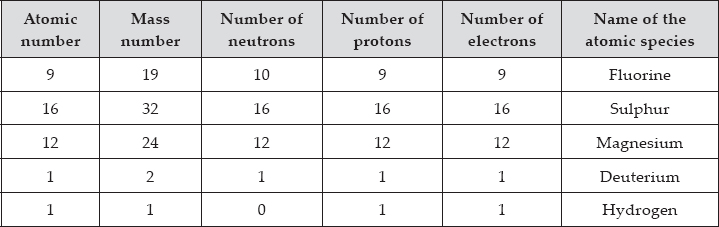# Structure of the Atom - NCERT Questions

Q 1.

What are canal rays?

SOLUTION:

The beam of rays which travel in a direction away from anode towards cathode when a gas taken in a discharge tube is subjected to the action of high voltage under low pressure are known as canal rays. It is also called anode rays. It was discovered by E. Goldstein in 1886.

Q 2.

If an atom contains one electron and one proton, will it carry any charge or not?

SOLUTION:

No, the atom will not carry any charge because electron has negative charge (–1) and proton has positive charge (+1). They neutralise each other.

Q 3.

On the basis of Thomson’s model of an atom, explain how the atom is neutral as a whole.

SOLUTION:

According to Thomson’s model of an atom :
A. An atom consists of a positively charged sphere and the electrons are embedded like the seeds in a water-melon.
B. The negative and positive charges are equal in magnitude. So, the atom as a whole is electrically neutral.

Q 4.

On the basis of Rutherford’s model of an atom, which sub-atomic particle is present in the nucleus of an atom?

SOLUTION:

Proton, positively charged sub-atomic particle is present in the nucleus of an atom.

Q 5.

Draw a sketch of Bohr’s model of an atom with three shells.

SOLUTION: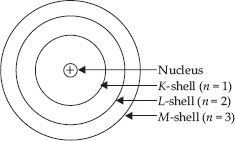Q 6.

What do you think would be the observation if the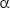-
particle scattering experiment is carried out using a foil of a metal other than gold?

SOLUTION:

On using the foil of heavy metal like gold (e.g., platinum, silver etc.) the observation will be same but if the foil is of light metal (e.g., sodium, magnesium etc.), the massive-particles may push the nucleus aside and may not be deflected back.

Q 7.

Name the three sub-atomic particles of an atom.

SOLUTION:

The three sub-atomic particles of an atom are : (A) electron (B) proton (C) neutron.

Q 8.

Helium atom has an atomic mass of 4 u and two protons in its nucleus. How many neutrons does it have?

SOLUTION:

Mass number of helium = 4
Number of protons = 2
Number of neutrons (n)
= Mass number (A) – No. of protons (p)
= 4 – 2 = 2
Thus, no. of neutrons = 2

Q 9.

Write the distribution of electrons in carbon and sodium atoms.

SOLUTION: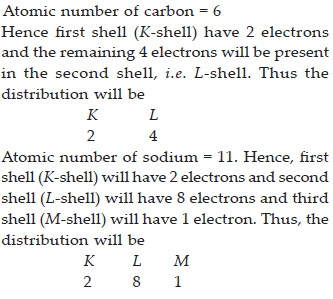Q 10.

If K and L shells of an atom are full, then what would be the total number of electrons in the atom?

SOLUTION:

The maximum number of electrons present in a shell = 2n2
where n = shell number
Value of n for K shell = 1
∴ Maximum number of electrons in K shell
= 2n2 = 2(1)2 = 2
Value of n for L shell= 2
∴ Maximum number of electrons in L shell
= 2(2)2 = 8
Thus, total no. of electrons = 2 + 8 = 10

Q 11.

How will you find the valency of chlorine, sulphur and magnesium?

SOLUTION:

Valency of an atom is the number of electrons gained, lost or shared so as to complete the octet of electrons in the valence shell.
Valency of chlorine: It has electronic configuration = 2, 8, 7
Thus, one electron is gained to complete its octet and so its valency is 1.
Valency of sulphur: It has electronic configuration = 2, 8, 6
Thus, two electrons are gained to complete its octet and hence its valency = 2
Valency of magnesium : It has electronic configuration = 2, 8, 2
Thus, it can lose two electrons to attain octet and hence its valency = 2

Q 12.

If number of electrons in an atom is 8 and number of protons is also 8, then (i) what is the atomic number of the atom and (ii) what is the charge on the atom?

SOLUTION:

We know that,
No. of electrons (e) = No. of protons (p)
= Atomic number (Z)
(I) No. of electrons = 8
∴ Atomic number = 8
(ii) The number of protons is equal to the number of electrons. So the atom will be neutral.

Q 13.

Atomic numbers of oxygen and sulphur are 8 and 16 respectively. Oxygen atom has 6 neutrons and sulphur atom has 16 neutrons. Find out the mass number of oxygen and sulphur atom.

SOLUTION:

Mass number of oxygen atom
= No. of protons + No. of neutrons
= 8 + 6 = 14
Mass number of sulphur atom
= No. of protons + No. of neutrons
= 16 + 16 = 32

Q 14.

Write the electronic configuration of any one pair of isotopes and isobars.

SOLUTION:

Isotopes of chlorine :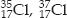Electronic configuration of each of them : 2, 8, 7
Isobars :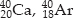Electronic configuration of 20Ca → 2, 8, 8, 2
Electronic configuration of 18Ar → 2, 8, 8

Q 15.

For the symbol H, D and T tabulate three sub-atomic particles found in each of them.

SOLUTION: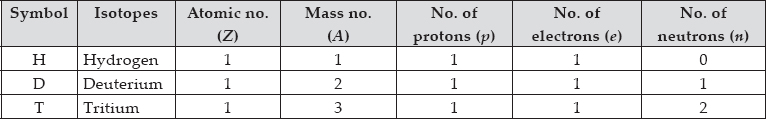Q 16.

Compare the properties of electrons, protons and neutrons.

SOLUTION: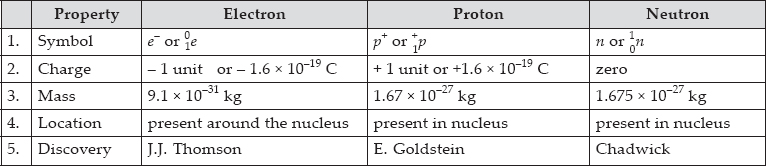Q 17.

What are the limitations of J.J. Thomson’s model of the atom?

SOLUTION:

The limitations of J.J Thomson’s model of an atom are following :
A. It could not explain the result of the scattering experiment performed by Rutherford.
B. It did not have any experimental evidence in its support.

Q 18.

What are the limitations of Rutherford’s model of the atom?

SOLUTION:

The limitations of Rutherford’s model of the atom are following :
A. It does not explain the stability of atom.
B. It does not explain the spectrum of hydrogen and other atoms.

Q 19.

Describe Bohr’s model of the atom.

SOLUTION:

The Danish physicist Neils Bohr proposed the following postulates for revising the Rutherford’s model.
A. Atom has central nucleus surrounded by electrons.
B. An atom consists of small heavy positively charged nucleus in the centre and the electrons revolve around it, in circular paths called orbits or shells..
C. Each orbit has fixed energy, so these orbits are called energy levels or energy shells.
D. The order of the energy of these energy shells will be
K < L < M < N < O < .........
or, 1 < 2 < 3 < 4 < 5 < .........
E. As long as an electron remains in a particular orbit, it does not lose or gain energy.
F. Energy is neither absorbed nor emitted when electron is moving in an orbit. But energy is absorbed when it jumps from lower orbit to higher orbit. Whereas energy is emitted when it jumps from higher orbit to lower orbit.

Q 20.

Compare all the proposed models of an atom given in this chapter.

SOLUTION: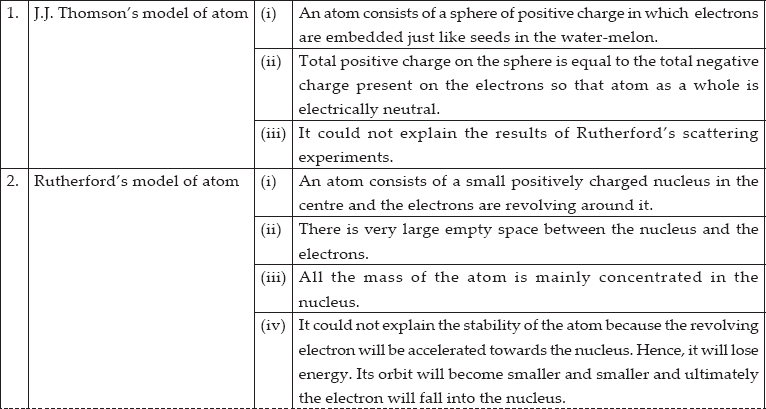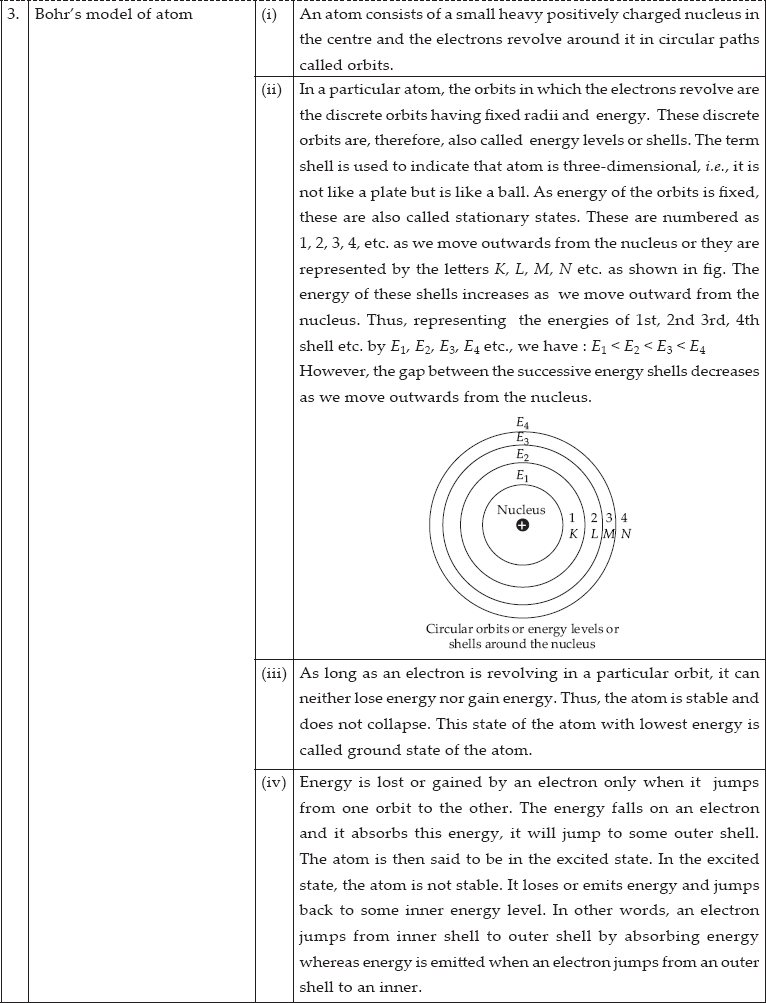Q 21.

Summarise the rules for writing the distribution of electrons in various shells for the first eighteen elements.

SOLUTION:

The distribution of elements in different orbits is governed by a scheme called Bohr-Bury scheme. There are following rules :
(I) The maximum number of electrons present in any shell is given by the formula 2n2. Where n = no. of orbit.
(ii) The maximum number of electrons that can be accommodated in the outermost shell is 8.
(iii) Electrons in an atom do not occupy a new shell unless all the inner shells are completely filled.

Q 22.

Define valency by taking examples of silicon and oxygen.

SOLUTION:

The number of electrons gained, lost or shared so as to complete the octet of electrons in valence shell is called valency.
Valency of silicon : It has electronic configuration → 2, 8, 4
Thus, 4 electrons are shared with other atoms to complete the octet and so its valency = 4
Valency of oxygen : It has electronic configuration → 2, 6
Thus, It will gain 2 electrons to complete its octet. So its valency = 2

Q 23.

Explain with examples (I) Atomic number, (ii) Mass number, (iii) Isotopes and (iv) Isobars. Give any two uses of isotopes.

SOLUTION:

(I) Atomic number : The number of protons present in the nucleus of an atom is called atomic number. It is denoted by Z. e.g., 4020Ca i.e., atomic number = 20
(ii) Mass number : The sum of the number of protons and neutrons present in the nucleus of an atom is called mass number. It is denoted by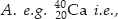mass number = 40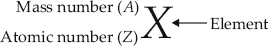(iii) Isotopes : The atoms of the same elements having same atomic number but different mass numbers are called isotopes.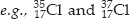(iv) Isobars : The atoms of the different elements having same mass number but different atomic numbers are called isobars.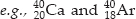Uses of isotopes : (I) As nuclear fuel : An isotope of uranium (U – 235) is used as a nuclear fuel.
(ii) In medical field : An isotope of cobalt is used in the treatment of cancer.

Q 24.

Na+ has completely filled K and L shells. Explain.

SOLUTION:

Atomic number of sodium (Na) = 11
No. of electrons in Na = 11
No. of electrons in Na+ = 11 – 1 = 10
Electronic configuration of Na+ →2, 8
K L
Thus, in Na+, K and L shells are completely filled.

Q 25.

If bromine atom is available in the form of, say two isotopes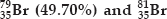(50.30%), calculate the average atomic mass of bromine atom.

SOLUTION: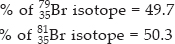% of 8135Br isotope = 50.3
Average atomic mass of bromine atom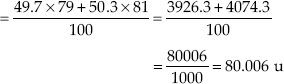Q 26.

The average atomic mass of sample of an element X is 16.2 u. What are the percentages of isotopesin the sample?

SOLUTION:

Let the % of isotope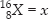and % of isotope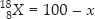Average atomic mass of an element (X) = 16.2
Average atomic mass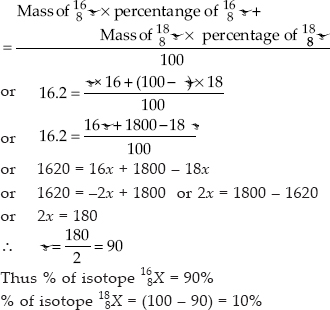Q 27.

If Z = 3, what would be the valency of the element? Also, name the element.

SOLUTION:

Ringworm (tinea) is a fungal infection of the skin, the scalp, or the nails. Ringworm is caused by the dermatophyte fungi-species of Microsporum and Epidermophyton and also affects animals, a source of infection for humans.

Q 28.

Composition of the nuclei of two atomic species X and Y are given as under :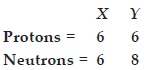Give the mass numbers of X and Y. What is the relation between the two species?

SOLUTION:

Mass number of X = No. of protons + No. of neutrons
= 6 + 6 = 12
Mass number of Y = 6 + 8 = 14
The species X and Y are the isotopes because their atomic numbers are same and their mass numbers are different i.e.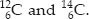Q 29.

For the following statements, write T for True and F for False.
(A) J.J. Thomson proposed that the nucleus of an atom contains only nucleons.
(B) A neutron is formed by an electron and a proton combining together. Therefore, it is neutral.
(C) The mass of an electron is about 1/2000 times that of proton.
(D) An isotope of iodine is used for making tincture iodine, which is used as a medicine.

SOLUTION:

(A) F : Because it was not proposed by J.J. Thomson.
(B) F : Because neutron is an independent sub-atomic particle.
(C) T : Because it is a fact known from experiments.
(D) F : Because tincture iodine is a solution of ordinary iodine in alcohol.

Q 30.

Rutherford’s alpha-particle scattering experiment was responsible for the discovery of
(A) atomic nucleus
(B) electron
(C) proton
(D) neutron

SOLUTION:

atomic nucleus

Q 31.

Isotopes of an element have
(A) the same physical properties
(B) different chemical properties
(C) different number of neutrons
(D) different atomic numbers

SOLUTION:

different number of neutrons

Q 32.

Number of valence electrons in Cl ion are
(A) 16
(B) 8
(C) 17
(D) 18

SOLUTION:

Electronic configuration of Cl(Z = 17) = 2, 8, 7. Thus, it has 7 valence electrons. Cl gains 1 electron to form Cl ion. So, number of valence electrons = 7 + 1 = 8.

Q 33.

Which one of the following is a correct electronic configuration of sodium?
(A) 2, 8
(B) 8, 2, 1
(C) 2, 1, 8
(D) 2, 8, 1

SOLUTION:

Atomic number of sodium (Na) = 11
Its electronic configuration = 2, 8, 1
Thus, (D) is correct.

Q 34.

Complete the following table.

SOLUTION:

First Line : Mass number = Atomic number (9) + No. of neutrons (10) = 19
No. of protons = Atomic number = 9
No. of electrons = Atomic number = 9
Name of the species = Fluorine (F)
Second Line : No. of neutrons = Mass number (32) – Atomic number (16) = 16
No. of protons = Atomic number = 16
No. of electrons = Atomic number = 16
Third Line : Atomic number = No. of protons = 12
No. of neutrons = Mass number (24) – Atomic number (12) = 12
No. of electrons = Atomic number = 12
Name of the species = Magnesium (Mg)
Fourth Line : Atomic number = No. of protons = 1
No. of neutrons = Mass number (2) – Atomic number (1) = 1
No. of electrons = Atomic number = 1
Name of the species = Deuterium (D).
Fifth Line : Atomic number = No. of protons = 1
Name of the species = Protium or Hydrogen (H)
The complete table can also be represented as :